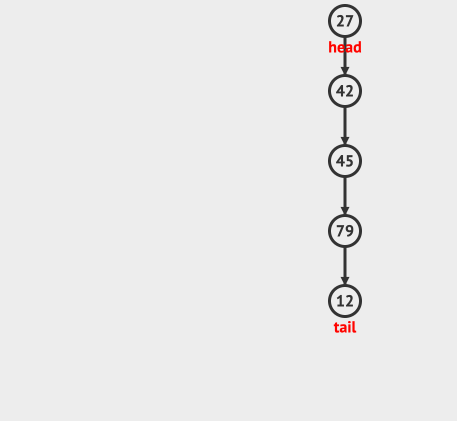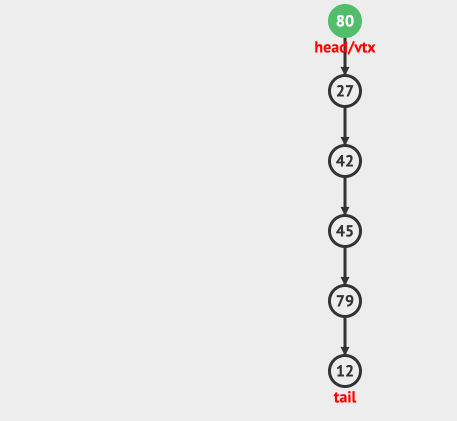# 栈的构成# 栈的实现

## 使用数组来实现栈

``````public class ArrayStack {

//实际存储数据的数组
private int[] array;
//stack的容量
private int capacity;
//stack头部指针的位置
private int topIndex;

public ArrayStack(int capacity){
this.capacity= capacity;
array = new int[capacity];
//默认情况下topIndex是-1，表示stack是空
topIndex=-1;
}

/**
* stack 是否为空
* @return
*/
public boolean isEmpty(){
}

/**
* stack 是否满了
* @return
*/
public boolean isFull(){
}

public void push(int data){
if(isFull()){
System.out.println("Stack已经满了，禁止插入");
}else{
array[++topIndex]=data;
}
}

public int pop(){
if(isEmpty()){
System.out.println("Stack是空的");
return -1;
}else{
return array[topIndex--];
}
}
}
``````

## 使用动态数组来实现栈

``````public void push(int data){
if(isFull()){
System.out.println("Stack已经满了，stack扩容");
expandStack();
}
array[++topIndex]=data;
}

//扩容stack，这里我们简单的使用倍增方式
private void expandStack(){
int[] expandedArray = new int[capacity* 2];
System.arraycopy(array,0, expandedArray,0, capacity);
capacity= capacity*2;
array= expandedArray;
}
``````

## 使用链表来实现

``````public class LinkedListStack {

class Node {
int data;
Node next;
//Node的构造函数
Node(int d) {
data = d;
}
}

public void push(int data){
}else{
Node newNode= new Node(data);
}
}

public int top(){
return -1;
}else{
}
}

public int pop(){
System.out.println("Stack是空的");
return -1;
}else{
return data;
}
}

public boolean isEmpty(){